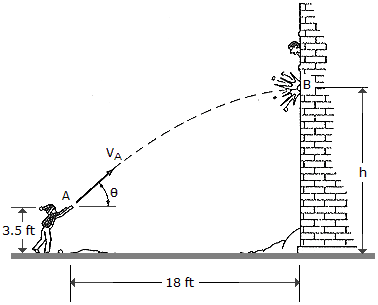# Engineering Mechanics - Kinematics of Particle (KOP) - Discussion

### Discussion :: Kinematics of Particle (KOP) - General Questions (Q.No.31)

31.The boy throws a snowball such that it strikes the wall of the building at the maximum height of its trajectory. If it takes t = 1.5 s to travel from A to B, determine the velocity vA at which it was thrown, the angle of release, and the height h.

 [A]. vA = 12.00 ft/s,= 24.4E, h = 21.7 ft [B]. vA = 49.8 ft/s,= 76.0E, h = 39.7 ft [C]. vA = 36.3 ft/s,= 24.4E, h = 18.2 ft [D]. vA = 48.3 ft/s,= 65.6E, h = 39.7 ft

Explanation:

No answer description available for this question.